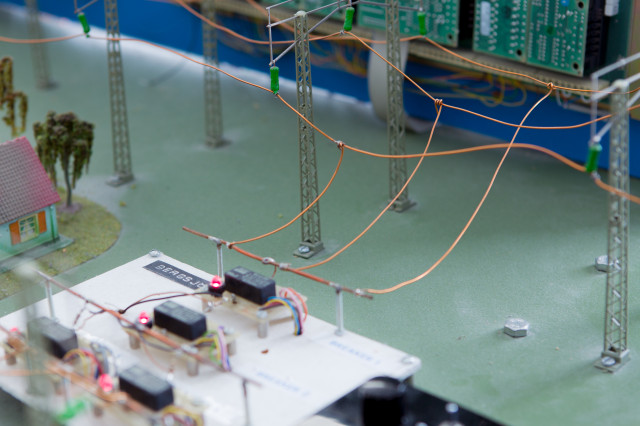The course gives a broad overview of modeling using stochastic processes in electrical engineering applications. Formulating problems using mathematical modeling is an important part of the course. Basics about continuous time an discrete time stochastic processes, especially weakly stationary processes. Definitions of probability distribution and density functions, statistical mean, mean power, variance, autocorrelation function, power spectral density, Gaussian processes and white noise. Linear filtering of stochastic processes, Ergodicity: Estimation of statistical properties from measurements. Sampling and reconstruction: Transformations between continuous and discrete time signals. Influence of sampling, sampling theorem. Pulse amplitude modulation. Errrors in the reconstruction of stochastic signals. Estimation theory: Linear estimation, orthogonality conditions. Prediction and Wiener filtering. Model based signal processing: Linear signal models, AR-models. Spectral estimation. Application of the above to simpler electrical engineering applications.

Course offering missing for current semester as well as for previous and coming semesters

## Content and learning outcomes

### Course contents

The course gives a broad overview of modeling using stochastic processes in electrical engineering applications. Formulating problems using mathematical modeling is an important part of the course.

Basics about continuous time an discrete time stochastic processes, especially weakly stationary processes. Definitions of probability distribution and density functions, statistical mean, mean power, variance, autocorrelation function, power spectral density,

Gaussian processes and white noise. Linear filtering of stochastic processes, Ergodicity: Estimation of statistical properties from measurements. Sampling and reconstruction: Transformations between continuous and discrete time signals. Influence of sampling, sampling theorem. Pulse amplitude modulation. Errrors in the reconstruction of stochastic signals. Estimation theory: Linear estimation, orthogonality conditions. Prediction and Wiener filtering. Model based signal processing: Linear signal models, AR-models. Spectral estimation. Application of the above to simpler electrical engineering applications.

### Intended learning outcomes

After passing the course you should be able to

• Analyze given problems regarding properties of weakly stationary stochastic processes.
• Analyze given problems in at least one of the areas filtering, sampling and reconstruction of weakly stationary processes.
• Analyze given problems in estimation and/or optimal filtering.
• Apply mathematical modeling tools to problems in electrical engineering. Develop simple software codes using, e.g., Matlab, and use this to simulate and analyze problems in the area. Report the methodology and results.
• Use a given mathematical model, or formulate one on your own, to solve a given technical problem in the area, analyze the result and justify if it is reasonable.

If you are passing the course with higher grades, you should, in addition to the above, be able to

• Analyze given problems in filtering, sampling and reconstruction of weakly stationary processes.
• Analyze given problems in estimation and optimal filtering.
• Formulate mathematical models which are applicable and relevant to a given problem formulation within the area. When vital information is missing, you should be able to judge and compare different possibilities as well as make reasonable assumptions to achieve a satisfactorily modeling performance.
• Use a given mathematical model, or one formulated by yourself, to solve a problem in the area; e.g., a problem composed of several interacting sub-problems or other problems requiring a more complex modeling, analyze the result and its validity.

### Course Disposition

No information inserted

## Literature and preparations

### Specific prerequisites

For single course students: General admission requirements, 120 credits and documented proficiency in English B or equivalent

### Recommended prerequisites

EQ1100 Signals and systems II, or equivalent
SF1901 Probability Theory and Statistics, or equivalent
EL1150 Introductory Matlab Course, or equivalent.

### Equipment

No information inserted

### Literature

Händel, Ottoson, Hjalmarsson, ”Signal Theory”, Third edition.

## Examination and completion

If the course is discontinued, students may request to be examined during the following two academic years.

A, B, C, D, E, FX, F

### Examination

• PRO1 - Project, 1,0 hp, betygsskala: P, F
• PRO2 - Project, 1,0 hp, betygsskala: P, F
• TEN1 - Examination, 5,5 hp, betygsskala: A, B, C, D, E, FX, F

Based on recommendation from KTH’s coordinator for disabilities, the examiner will decide how to adapt an examination for students with documented disability.

The examiner may apply another examination format when re-examining individual students.

### Other requirements for final grade

Written exam, (TEN1; 5.5 ECTS credits; Grading: A-F).
Project assignment 1 and 2 (LAB1; 1 ECTS credits LAB2; 1 ECTS credits; Grading: Pass/Fail).

### Opportunity to complete the requirements via supplementary examination

No information inserted

### Opportunity to raise an approved grade via renewed examination

No information inserted

### Examiner

No information inserted

### Ethical approach

• All members of a group are responsible for the group's work.
• In any assessment, every student shall honestly disclose any help received and sources used.
• In an oral assessment, every student shall be able to present and answer questions about the entire assignment and solution.

## Further information

### Course web

Further information about the course can be found on the Course web at the link below. Information on the Course web will later be moved to this site.

Course web EQ1240

### Offered by

EES/Signal Processing

### Main field of study

Electrical Engineering, Technology

First cycle